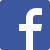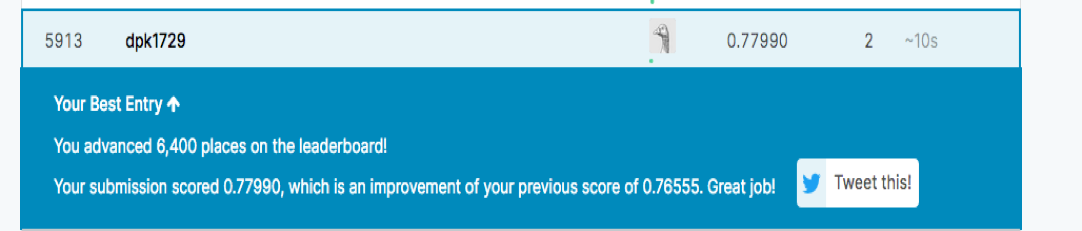Here is the latest from Julia Computing
BLG# Automatic Differentiation Meets Conventional Machine Learning

24 Feb 2020 | Deepak Suresh

Differentiation is a central problem in many fields including deep learning, finance, scientific computing and others. The two conventional techniques used include:

• Symbolic differentiation which can result in complex and redundant expressions

• Numerical differentiation which can lead to large numerical errors

Automatic differentiation (AD) has been behind recent advances in deep learning. A Differentiable Programming System to Bridge Machine Learning and Scientific Computing, makes AD a first class feature in Julia language , helps differentiate programs, rather than simple network architectures.

Julia helps bring AD to the world of machine learning beyond neural networks. We will look at a specific use case and see how Julia allows us to compose functionalities from multiple packages seamlessly. (JuliaLang: The Ingredients for a Composable Programming Language)

Introduction

One of the advantages of Julia is its composability. Automatic differentiation can be applied in a conventional machine learning tool like xgboost. These two packages were developed independently yet they work together seamlessly. In order to illustrate this let’s look at how easily custom loss functions can be implemented in XGBoost with Zygote.

There are a lot of in-built loss functions in xgboost, but these may be suboptimal for a lot of real world problems. Consider testing for a rare disease. False positives are not good, but they are not costly mistakes in the sense that further tests can easily rule them out. On the other hand false negatives are far worse than a false positive. A person who has a condition and needs immediate care has been incorrectly identified as healthy. False negatives are far more costly than false positives. These kinds of scenarios are common in real world machine learning problems. This is where custom loss functions are useful.

The default loss functions available off-the-shelf might not be optimal for the business objectives. On the other hand, a custom loss function that closely matches the business objectives can take the asymmetry on errors into account. In the disease detection scenario discussed above, false negative errors are far more costly than false positives. Using a custom loss function that penalizes the model heavily for making false negative errors will result in a model that is averse to false negatives.

Problem formulation

We have chosen to predict the survival chances on the Titanic ocean liner using a supervised ML technique called XGBoost. It is an implementation of gradient boosting where an ensemble of weak decision tree learners are combined to produce a strong model.

In order to deal with asymmetric penalties, like the case of disease detection that was discussed above, XGBoost permits custom loss functions. First we build a simple baseline model. Let’s see how we do this in Julia.

We use CSVFiles to load the training data, and DataFrames formanipulation.

julia> using CSVFiles, DataFrames


The names method will give the features in this dataframe.

  julia> names(df)
12-element Array{Symbol,1}:
:PassengerId
:Survived
:Pclass
:Name
:Sex
:Age
:SibSp
:Parch
:Ticket
:Fare
:Cabin
:Embarked


Survived is the target variable, we will have to clean and process the rest of the features before a model can be built on it. We will be building a simple model with a few features, which are Age, Embarked, Sex, Pclass, SibSp, Parch, Fare

Data preprocessing

We will have to clean up the data a bit. The following line gives the count of number of missing values in column Embarked

julia> sum(df[:,:Embarked] .== "")
2


XGBoost does support learning on missing values, but in this case there are only 2 of them. So, it is better that we impute them with the most frequent value in the column.

Replace missing values with the most frequent port:

julia> df[df[:,:Embarked] .== "", :Embarked] = "S"


The column Age also has missing values, the following command tells us that there are 177 rows in the Age column with missing values

julia> sum(ismissing.(df[:Age]))
177


Age being a numerical feature, it is better that we use the average value of Age column to impute the missing values

Replace missing values with the average:

julia> using Statistics
julia> average_age = mean(df[.!ismissing.(df[:Age]), :Age])
julia> df[ismissing.(df[:Age]), :Age] = average_age


We can use one-hot encoding for categorical features such as Pclass and Embarked. One-hot encoding creates a binary feature for each value of the categorical feature. For instance, the feature Embarked has 3 fields, viz. S, C, Q One hot encoding will create 3 binary features, each corresponding to one of the unique values of the categorical column. The binary feature will Embarked will have 1s in rows where the value of Embarked was S, and 0s everywhere in other rows.

julia>for i in unique(df.Pclass)
df[:,Symbol("Pclass_"*string(i))] = Int.(df.Pclass .== i)
end

julia>for i in unique(df.Embarked)
df[:,Symbol("Embarked_"*string(i))] = Int.(df.Embarked .== i)
end


Gender can be encoded as a binary feature:

julia> gender_dict = Dict("male"=>1, "female"=>0);
julia> df[:Sex] = map(x->gender_dict[x], df[:Sex]);


Building our baseline model

Let’s split the dataset into training and validation set. The training set can be created as below

julia> x_train = convert(Matrix{Float32},select(df[1:800,:],Not(:Survived)))
julia> y_train = convert(Array{Float32}, df[1:800,:Survived])


Validation set:

julia> x_val = convert(Matrix{Float32},select(df[801:end,:],Not(:Survived)))
julia> y_val = convert(Array{Float32}, df[801:end,:Survived])


Create a DMatrix:

julia> train_dmat = DMatrix(x_train, label=y_train)


Train the model:

julia> bst_base = xgboost(train_dmat,2, eta=0.3, objective="binary:logistic", eval_metric="auc")
 train-auc:0.893250
 train-auc:0.899080


Get predictions on the validation set:

julia> ŷ = predict(bst, x_val)


The following function will calculate the accuracy and weighted f score:

function evaluate(y, ŷ;threshold=0.5)
out = zeros(Int64, 2,2)
ŷ = Int.(ŷ.>=threshold)
out[1,1]=sum((y.==0).&(ŷ.==0))
out[2,2]=sum((y.==1).&(ŷ.==1))
out[2,1]=sum((y.==1).&(ŷ.==0))
out[1,2]=sum((y.==0).&(ŷ.==1)
r0 = out[1,1]/(out[1,1]+out[1,2])
p0 = out[1,1]/(out[1,1]+out[2,1])
f0 = 2*p0*r0/(p0+r0
r1 = out[2,2]/(out[2,2]+out[2,1]
p1 = out[2,2]/(out[2,2]+out[1,2]
f1 = 2*r1*p1/(p1+r1
println("Weighted f1 = ", round((sum(y .== 0.0)/length(y)) * f0 + (sum(y .== 1.0)/length(y)) * f1	digits=3))
println("Accuracy =", (out[2,2]+out[1,1])/sum(out))
out
end


Let’s look at the performance of the baseline model:

julia> evaluate(y_val, ŷ)
Weighted f1 = 0.845
Accuracy = 0.8461538461538461
2×2 Array{Int64,2}:
51 6
8 26


Let’s submit this to Kaggle to get a tangible measure of the model performance. The current model is at 12300^th^ position on the leaderboardCustom loss function

There are 8 false negatives, in order to reduce false negatives, we can weigh false negatives higher than false positives in our loss function.

The signature of custom loss should be:

function weighted_loss(preds::Vector{Float32}, dtrain::DMatrix)
hessians = … #calculate hessians
end


The conventional approach is to calculate the gradients and Hessians by hand and then translate them to code. Let’s start by looking at the log loss function used by the model.

Notice that the term -yln(sigma(x)) which penalizes false negatives has a coefficient of 1.

We can change this weight to make the model penalize false negatives more than false positives. With a weight w on false positives the equation will look like this:

XGBoost admits loss functions in the signature that we defined above. We will have to find the gradient and Hessian of this loss and plug them in the function.

The gradient turns out to be:

Differentiating the above again gives us the second derivative:

Now we can complete our function with a slightly higher penalty, i.e. 1.5:

function weighted_loss(preds::Vector{Float32}, dtrain::DMatrix)
beta = 1.5
p = 1. ./ (1 .+ exp.(-preds))
grad = p .* ((beta .- 1) .* y .+ 1) .- beta .* y
hess = ((beta .- 1) .* y .+ 1) .* p .* (1.0 .- p)
end


Note that this required us to do some cumbersome calculus to implement the custom loss. A smarter approach would be to invoke Julia’s automatic differentiation to calculate gradients and Hessians. Apart from not having to do the math, it gives us flexibility as well since a slight change in the function means we won’t have to redo the math again.

Automatic differentiation

XGBoost outputs scores that need to be passed through a sigmoid function. We do this inside the custom loss function that we defined above. Let’s define it here explicitly:

σ(x) = 1/(1+exp(-x))


The weighted log loss can be defined as:

weighted_logistic_loss(x,y) = -1.5 .* y*log(σ(x)) -1 .* (1-y)*log(1-σ(x))


We have added a weight of 1.5 to false negatives.

gradient_logistic(x,y) = gradient(weighted_logistic_loss,x,y)


The gradient method differentiates the weighted_logistic_loss function. The parameters of the weighted_logistic_loss function are also passed alongside. We then take the first element with  because we are interested in the derivative with respect to the first parameter x.

Similarly, the Hessian can be calculated by differentiating the above gradient function:

hess_logistic(x,y) = gradient(grad_logistic,x,y)


We can define the custom loss function using this gradient and Hessian:

function custom_objective(preds::Vector{Float32}, dtrain::DMatrix)
y = get_info(dtrain, "label")
hess = hess_logistic.(preds,y)
end


This can be passed to the XGBoost trainer:

julia> bst = xgboost(train_dmat, 2,eta=0.3, eval_metric="auc", obj=custom_objective)
 train-auc:0.892897
 train-auc:0.899565


At this point we can evaluate the model, and compare it against the baseline:

julia> ŷ = predict(bst, x_val)
julia> evaluate(y_val, ŷ)
Weighted f1 = 0.878
Accuracy =0.8791208791208791
2×2 Array{Int64,2}:
53 4
7 27


That’s quite an improvement for a small change in the loss function. We didn’t have to do any of the taxing math to try out this objective function. All we had to do was define the function and let Zygote take care of calculating the gradient and Hessian.

At this point, we can submit the results from the new model to Kaggle. We have been able to move 6400 places up on the leaderboard with very little effort.This exercise illustrates the composability of Julia packages and the flexibility that it gives data scientists.

## Recent posts

Grokking Deep Learning with Julia
29 Jul 2020 | Deepak Suresh